# Civil Engineering - GATE Exam Questions - Discussion

### Discussion :: GATE Exam Questions - Section 1 (Q.No.26)

26.

A cantilever beam of length L and a cross section with shape factor f supports a concentrated load P as shown below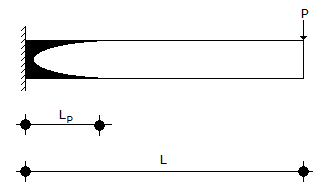The length LP of the plastic zone, when the maximum bending moment equals the plastic moment MP, given by

 [A].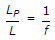[B].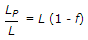[C].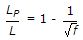[D].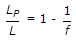Explanation:

No answer description available for this question.

 Ashish said: (Jan 11, 2015) Option D is correct. Mp/M = f = L/(L-Lp). Lp/L = 1-1/f.

 Vyshnavi said: (Mar 25, 2015) If f = L/(L-LP) , then f = 1-(LP/L) OR LP/L = f-1. But how LP/L = 1-1/f ? Please explain in detail.

 Chad said: (Aug 6, 2015) @Vyshnavi, f=L/(L-Lp), then 1/f = (L-Lp)/L = 1-(Lp/L) not f = 1-(Lp/L) as you stated.

 Madhumanti said: (Sep 1, 2015) f = L/(L-Lp). fL-fLp = L. L(f-1) = fLp. Lp/L = (f-1)/f. Lp/L = 1-(1/f).

 Kirubakara Raj said: (Aug 16, 2016) Thank you very much for your explanations.

 Rohit said: (Sep 18, 2016) How f = L/L - Lp?

 Rahul said: (Nov 18, 2016) Thanks for explaining it.

 Anuz said: (Feb 10, 2018) f = L /(L-Lp). (1/f) = (L-Lp)/L. (1/f) = (L/L) - (Lp/L). (Lp/L) = 1 - (1/f ).

 Uttam said: (Mar 12, 2018) It is lp/l=1/f. Ref: Agor Book.

 Nilima said: (Jan 26, 2019) Thanks all for explaining it.